# Young's Modulus of Elasticity|Elastic Modulus

### 5. Elastic Modulus

• Stress required to produce a given strain in a material body depends on the nature of material under stress.
• We already know that ratio of stress to strain is known as elastic modulus of the material.
• Larger is the elastic modulus of a given material, greater would be the stress needed to produce a given strain.
• There are three different types of modulus of elasticity- Young's Modulus of elasticity, Bulk Modulus of elasticity and Modulus of Rigidity.
(a) Young's Modulus of Elasticity
• Young's Modulus of elasticity is the ratio of longitudinal stress to longitudinal strain.
• It is denoted by Y.
• Young's Modulus of elasticity is given by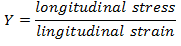or
• Let us now consider a wire of length l having area of cross-section equal to A. If the force F acting on the wire, stretches the wire by length Δ l then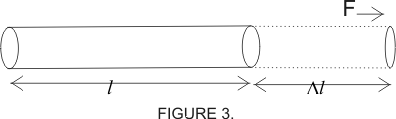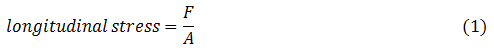and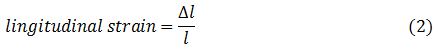From (1) and (2) we have Young's modulus of elasticity as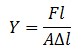• Young's modulus of elasticity has dimensions of force/Area i.e. of pressure.
• Unit of Young's modulus is N/m2.
• If area of cross-section of a wire is given by A = πr2 then Young's modulus is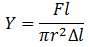again if A = π r2 = 1cm2 and Δ l = l = 1cm then
Y = F
Thus, Young's modulus can also be defined as the force required to double the length of a wire of unit length and unit area of cross-section.
This is a short video on experimental measurement of Young's Modulus of a given wire. This video is developed by CDAC Mumbai & Amrita University under research grant from Department of IT, Government of India
A measure of stiffness that is independent of the particular sample of a substance is the Young modulus E. The Young Modulus is named after Thomas Young .The Young Modulus is also known as Young's Modulus or the elastic modulus or tensile modulus. Young's Modulus can vary considerably depending on the exact composition of the material. (as stated in video description)

(b) Bulk Modulus of Elasticity
• The ratio of normal stress to volume strain within elastic limits is called Bulk Modulus of elasticity of a given material.
• It is denoted by K.
• Suppose a force F is applied normal to a surface of a body having cross-sectional area equal to A.
If applied force bring about a change ΔV in the volume of the body and V is the original volume of the body then,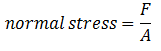and
Volume strain = $\frac{\Delta V}{V}$
So, Bulk Modulus of elasticity would be,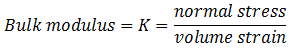Thus,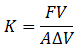• For gases and liquids the normal stress is caused by change in pressure i. e.,
normal stress = change in pressure ΔP.
Thus, bulk Modulus is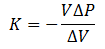here negative sign indicates that the volume decreases if pressure increases and vice-versa.
• For extremely small changes in pressure and volume, the Bulk Modulus is given by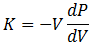• Reciprocal of Bulk Modulus is called compressibility of substance. Thus,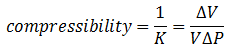(c) Modulus of Rigidity
• When a body is sheared, the ratio of tangential stress to the shearing strain within elastic limits is called the Modulus of Rigidity.
• If lower face of the rectangular block shown below in the figure, is fixed and tangential force is applied at the upper face of area A, then shape of rectangular block changes.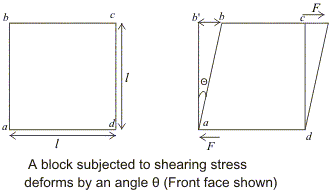So,
shearing strain = θ ≅ tanθ
or,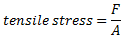Thus,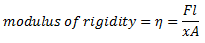Points you must note about elastic modulus
1. The value of elastic modulus is independent of stress and strain. It depends only on the nature of the material.
2. Greater value of modulus of elasticity means that the material has more elasticity i.e., material is more elastic.
3. Young's Modulus and Shear Modulus exists only for solids while Bulk Modulus is defined for all three stats of matter.
4. Three modulus of elasticity $Y$, $\eta$ and $K$ depends on temperature. Their value decreases with the increase in temperature.
5. In case of longitudinal stress , shape remains unchanged while the volume changes. In tensile one volume increases while in compressive one volume decreases.
6. In shear stress , volume remains the same but shape changes.
7. In volume stress , volume changes but shape remains the same.